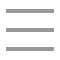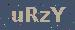个人随笔

leetcode11. 盛最多水的容器：双指针法
2021-09-06 12:29:27

### 解题2方法

``/**`` * 11. 盛最多水的容器`` * https://leetcode-cn.com/problems/container-with-most-water/`` * @author 爱吃鱼的乌贼`` *`` */``public class Leetcode11 {``    /**``     * 用双指针的方法，让两个指针分别指向第一个和最后一个值，因为能够剩多少水，靠的是较小的挡板，所以``     * 哪一个指针对应的小，就移动哪一个，毕竟移动较大的指针是没有意义的，因为两个指针的距离是变小的，容量又是``     * 小的挡板*距离，所以移动高的挡板，没有意义，不可能大于不移动的容量``     * @param height``     * @return``     */``    public int maxArea(int[] height) {``        int len = height.length;``        //开始下标``        int first = 0;``        //结束下标``        int last = len-1;``        //最大容量``        int max = 0;``        //如果开始下标小于结束下标，则进行移动判定``        while(first<last) {``            //1、获取下标之间的距离``            int t = last-first;``            //2、获取叫矮的挡板值``            int first_value = height[first];``            int last_value = height[last];``            int min = getMin(first_value, last_value);``            //3、获取当前的容量``            int h = t*min;``            if(h>max) {``                max=h;``            }``            //4、移动较小的下标``            if(first_value<last_value) {``                first++;``            }else {``                last--;``            }``        }``        return max;``    }``    public int getMin(int first,int second) {``        if(first>=second) {``            return second;``        }else {``            return first;``        }``    }``    public static void main(String[] args) {``        int[] height = new int[] {1,8,6,2,5,4,8,3,7};``        System.out.println(new Leetcode11().maxArea(height));``    }``}``

### 总结

57Copyright : 个人随笔   备案号 : 粤ICP备18099399号